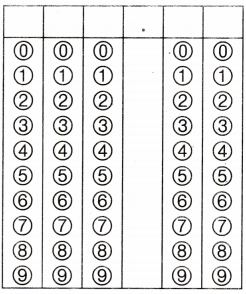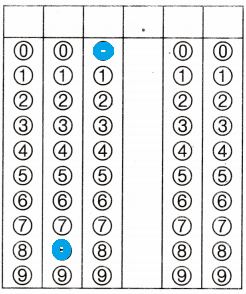Refer to our Texas Go Math Grade 4 Answer Key Pdf to score good marks in the exams. Test yourself by practicing the problems from Texas Go Math Grade 4 Module 9 Assessment Answer Key.

Vocabulary

• counting numbers
• compatible numbers
• remainder

Choose the best term from the box to complete the sentence.

Question 1.
Numbers that are easy to compute mentally are called
Compatible numbers
Explanation:
The numbers which are close to the actual numbers, and can be easily added, subtracted or divided orally are known as compatible numbers.”. These numbers are used for carrying out basic mathematical functions.
For example;
Compatible numbers for 232 and 11 are 240 and 12.

Question 2.
When a number cannot be divided evenly, the amount left over is called the _____________ .
Remainder
Explanation:
Remainder is the value left after the division.
If a number (dividend) is not completely divisible by another number ( divisor) then we are left with a value once the division is done.
For example, 10 is not exactly divided by 3.
Since the closest value we can get is 3 x 3 = 9, which is 1 less than 10.

Concepts and Skills

Use an array to find the quotient and remainder.

Question 3.
26 ÷ 6
Quotient = 4
Remainder = 2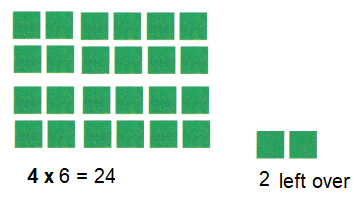Explanation:
A quotient is a result obtained in the division process.
Remainder is the value left after the division.

Question 4.
19 ÷ 4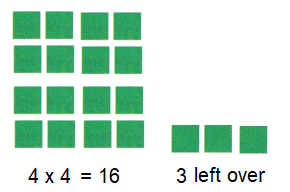Quotient = 4
Remainder = 2
Explanation:
A quotient is a result obtained in the division process.
Remainder is the value left after the division.

Use basic facts and place value to find the quotient.

Question 5.
810 ÷ 9 = _____________
Explanation:
810 ÷ 9,  we identify the basic fact 81 ÷ 9 = 9
we use place value 810  = 81 tens
we divide 81 tens ÷ 9 = 9 tens
= 90

Question 6.
210 ÷ 7 = _____________
Explanation:
210 ÷ 7,  we identify the basic fact 21 ÷ 7 = 3
we use place value 210  = 21 tens
we divide 21 tens ÷ 7 = 3 tens
= 30

Question 7.
3,000 ÷ 6 = _____________
Explanation:
3000 ÷ 6,  we identify the basic fact 3000 ÷ 6 = 500
we use place value 3000  = 30 hundreds
we divide 30 hundreds ÷ 6 = 5 hundreds
= 500

Use compatible numbers to estimate the quotient.

Question 8.
635 ÷ 9
Explanation:
635 compatible number is 630
630 ÷ 9 = 7
Quotient is 7 remainder = 0

Question 9.
412 ÷ 5
Explanation:
412 compatible number is 400
400 ÷ 5 = 20
Quotient is 20 remainder = 0

Question 10.
490 ÷ 8
Explanation:
490 is written as 480 compatible number
480 ÷ 8
Quotient is 6 remainder = 0

Use grid paper or base-ten blocks to model the quotient.
Then record the quotient.

Question 11.
63 ÷ 3 = _____________
Explanation:Question 12.
85 ÷ 5 = _____________
Explanation:Question 13.
168 ÷ 8 = _____________
Explanation: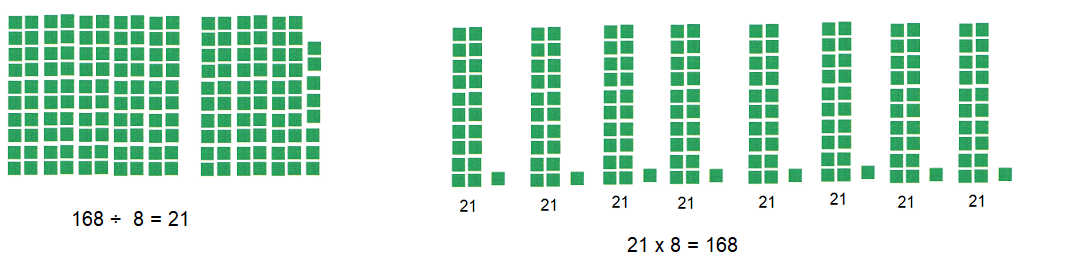Question 14.
Ana has 296 coins in her coin collection. She put the same number of coins in each of 5 jars and 2 boxes. About how many coins are in each jar and box?
Option(B)
Explanation:
Ana has 296 coins in her coin collection.
She put the same number of coins in each of 5 jars and 2 boxes.
5 + 2 = 7
Number of coins in each jar and box
296 ÷ 7 =42.28
Approximately 40 coins

Question 15.
There are 4 students on a team for a relay race. How many teams can be made from 27 students?
(A) 4
(B) 3
(C) 6
(D) 7
Option(B)
Explanation:
There are 4 students on a team for a relay race.
Number of teams can be made from 27 students
27 ÷ 4 = 3

Question 16.
A snack vendor had 400 bags of peanuts and 240 bags of popcorn. She sold the same number of bags of snacks at each of 8 baseball games. If she sold all her snacks, how many bags of snacks did she sell at each game?

Record your answer and fill in the hubbies on the grid. Be sure to use the correct place value.